You may also likeChocolate

There are three tables in a room with blocks of chocolate on each. Where would be the best place for each child in the class to sit if they came in one at a time?Four Triangles Puzzle

Cut four triangles from a square as shown in the picture. How many different shapes can you make by fitting the four triangles back together?Cut it Out

Can you dissect an equilateral triangle into 6 smaller ones? What number of smaller equilateral triangles is it NOT possible to dissect a larger equilateral triangle into?

Multiples Grid

Age 7 to 11 Challenge Level:

Here is a $100$ grid with some numbers shaded: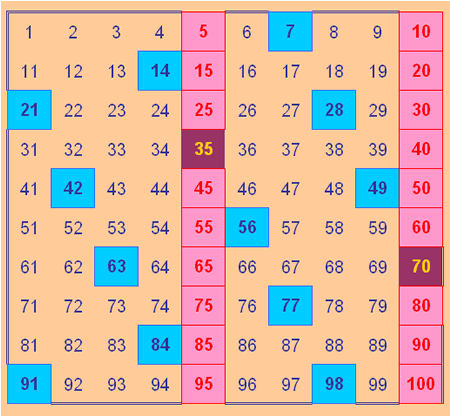What do all the numbers shaded blue have in common?
What do you notice about all the numbers shaded pink?
Can you work out why two of the numbers are shaded in a maroon colour?

Now, here is part of a $100$ square shaded in a different way: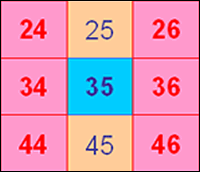Can you explain the shading this time?

Here are some more parts of the $100$ square, each one shaded according to different rules. Can you work out what the rules are for each?
Is there only one solution each time?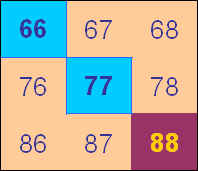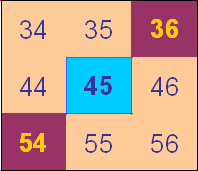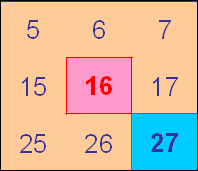This problem is featured in Maths Trails - Excel in Problem Solving, one of the books in the Maths Trails series written by members of the NRICH Team and published by Cambridge University Press. For more details about the other books in the series, please see our publications page .